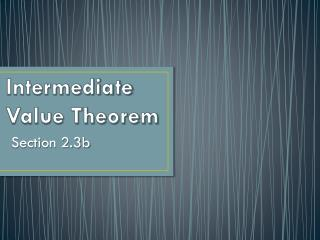# Intermediate Value Theorem - PowerPoint PPT PresentationDownload PresentationIntermediate Value Theorem

Intermediate Value TheoremDownload Presentation## Intermediate Value Theorem

- - - - - - - - - - - - - - - - - - - - - - - - - - - E N D - - - - - - - - - - - - - - - - - - - - - - - - - - -
##### Presentation Transcript

1. Intermediate Value Theorem Section 2.3b

2. Intermediate Value Property – describes a function that never takes on two values without taking on all the values in between. The Intermediate Value Theorem for Continuous Functions A function y = f (x) that is continuous on a closed interval [a, b] takes on every value between f (a) and f (b). In other words, if y is between f (a) and f (b), then y = f (c) for some c in [a, b]. 0 0 y x 0

3. Exploration 1: Removing a Discontinuity Let 1. Factor the denominator. What is the domain of f ? Domain: 2. Investigate the graph of f around x = 3 to see that f has a removable discontinuity at x= 3. It appears that the limit of f as x 3 exists and is a little more than 3. 3. How should fbe redefined at x = 3 to remove the discontinuity? Use zoom-in and tables as necessary. should be defined as

4. Exploration 1: Removing a Discontinuity Let 4. Show that (x – 3) is a factor of the numerator of f, and remove all common factors. Now compute the limit as x 3 of the reduced form for f. for Thus,

5. Exploration 1: Removing a Discontinuity Let 5. Show that the extended function is continuous at x = 3. The function g is the continuous extension of the original function f to include x = 3. so g is continuous at x = 3

6. Guided Practice Give a formula for the extended function that is continuous at the indicated point. For

7. Guided Practice Give a formula for the extended function that is continuous at the indicated point. The extended function:

8. Guided Practice Sketch a possible graph for a function f that has the properties stated below. exists, but does not. One possible answer: y x 3

9. Guided Practice Sketch a possible graph for a function f that has the properties stated below. exists, , but does not exist. y One possible answer: x –2

10. Guided Practice Sketch a possible graph for a function f that has the properties stated below. exists, exists, but f is not continuous at x = 4. One possible answer: y x 4

11. Guided Practice Sketch a possible graph for a function f that has the properties stated below. is continuous for all x except x = 1, where f has a non-removable discontinuity. One possible answer: y x x = 1

12. Guided Practice Find a value for a so that the given function is continuous. What might the graph look like? We require that Substitute: Does this answer make sense graphically?